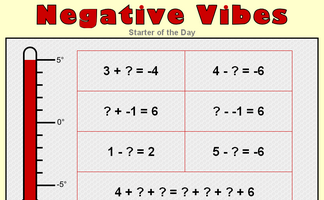#There are 366 different Starters of The Day, many to choose from. You will find below some starters on the topic of Negative Numbers. A lesson starter does not have to be on the same topic as the main part of the lesson or the topic of the previous lesson. It is often very useful to revise or explore other concepts by using a starter based on a totally different area of Mathematics.

Main Page

### Negative Numbers Starters:Copy and complete the multiplication grid. The higher levels include negative numbers.Perform calculations involving negative numbersPractise techniques for answering questions involving negative numbers.

## Exercises#### Bidmaze

Find your way through the maze encountering mathematical operations in the correct order to achieve the given total.#### Boxed In Numbers

The classic dots and boxes game with the addition of some positive and negative numbers which determine your score.#### Inequalities

Check that you know what inequality signs mean and how they are used to compare two quantities. Includes negative numbers, decimals, fractions and metric measures.#### Negative Magic

Four partially completed magic squares containing negative numbers. Fill in the missing numbers.#### Negative Numbers

Use negative numbers in basic arithmetic and algebraic calculations and word problems.#### Number Line

This number line visual aid is designed to be projected onto a whiteboard for whole class exposition.A self marking exercise on the reading of scales of different types.#### Temperatures

Test your understanding of negative numbers with this self marking quiz about temperatures.#### Think of a Number

Ten students think of a number then perform various operations on that number. You have to find what the original numbers were.

### Search

The activity you are looking for may have been classified in a different way from the way you were expecting. You can search the whole of Transum Maths by using the box below.

Have today's Starter of the Day as your default homepage. Copy the URL below then select
Tools > Internet Options (Internet Explorer) then paste the URL into the homepage field.

Set as your homepage (if you are using Internet Explorer)

Do you have any comments? It is always useful to receive feedback and helps make this free resource even more useful for those learning Mathematics anywhere in the world. Click here to enter your comments.For All: